# 1.背景介绍

• 超参数个数较多，训练前需要大量初始化，主要靠经验调整，使得DNN更像一门艺术而非科学（有人说深度学习就是在调参）；
• DNN的训练要求大量的训练数据，数据的标注成本太高；
• DNN是一个黑盒子，训练的结果很难去解释描述，并且学习行为很难用理论去分析解释；
• DNN在训练之前就得确定具体的网络结构（虽然dropout等的技术可以增加网络结构的一些不确定性），模型的复杂度也是提前设定好的。

Can deep learning be realized with non-differentiable modules?

• 深度学习是否就等同于DNN（深度模型是不是只能通过可微的函数结构去构建）？
• 是否可以不通过后向传播训练深度模型？
• 在一些RandomForest，XGBoost，lightGBM，catBoost成功的学习任务上,深度模型是否也能够战胜它们？

# 2.gcForest模型灵感来源

## 2.1 DNN中的灵感来源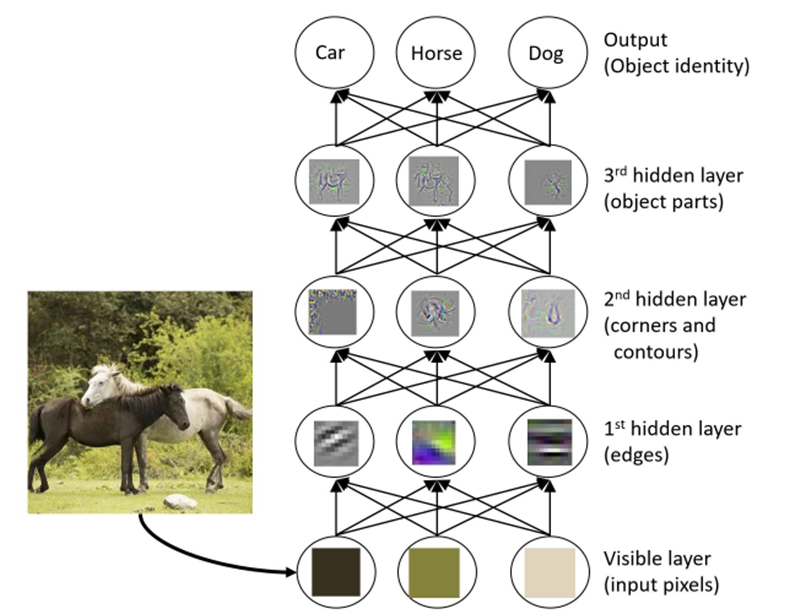• layer-by-layer processing
• in-model feature transformation
• suffcient model complexity

## 2.2 集成学习中的灵感来源

• 数据样本机制：通过产生不同的数据样本来训练子模型；
• 输入特征机制：通过选取不同特征子空间来训练子模型；
• 学习参数机制：通过设定不同参数来使得子模型具有多样性；
• 输出表示机制：通过不同的输出表示增加子模型的多样性；

# 3.gcForest模型

## 3.1 级联森林结构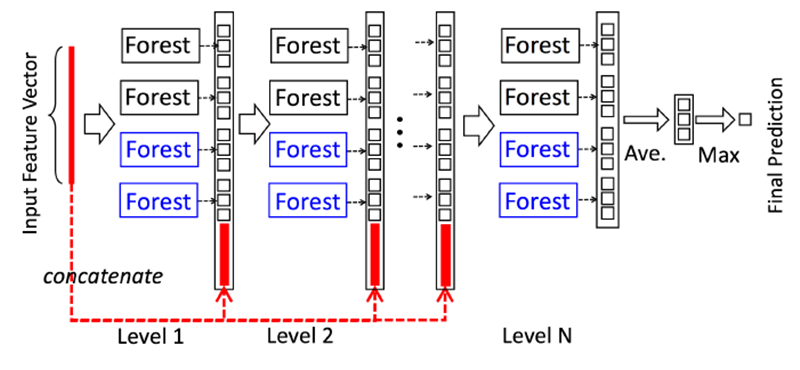• 每一个Level包含若干个集成学习的分类器（这里是决策树森林，你可以换成XGBoost，lightGBM，catBoost等），这是一种集成中的集成的结构；
• 为了体现多样性，黑色和蓝色的Forest代表了若干不同的集成学习器，为了说明这里用了两种，黑色的是完全随机森林：由500棵决策树组成，每棵树随机选取一个特征作为分裂树的分裂节点，然后一直生长，直到每个叶节点细分到只有一个类或者不多于10个样本，蓝色的表示普通的随机森林：由500棵决策树组成，每棵树通过随机选取sqrt(d)(d表示输入特征的维度)个候选特征然后通过gini系数筛选分裂节点；
• gcForest采用了DNN中的layer-by-layer结构，从前一层输入的数据和输出结果数据做concat作为下一层的输入；
• 为了防止过拟合的情况出现，每个森林的训练都使用了k-折交叉验证，也即每一个训练样本在Forest中都被使用k-1次，产生k-1个类别列表，取平均作为下一个Level级联的输入的一部分；
• 当级联扩展到新的Level后，通过验证集去评估之前所有级联结构的表现，如果评估结果没有太大的改变或提升则训练过程结束，因此级联结构的Level的个数被训练过程决定；
• 这里要注注意的是，当我们想控制训练过程的loss或限制计算资源的时候，我们也可以使用训练误差，而不是交叉验证的误差同样能够用于控制级联的增长；
• 以上这一点就是gcForest和神经网络的区别，gcForest可以通过训练自适应的调整模型的复杂度，并且在恰当的时候停止增加我们的Level；

## 3.2 多粒度扫描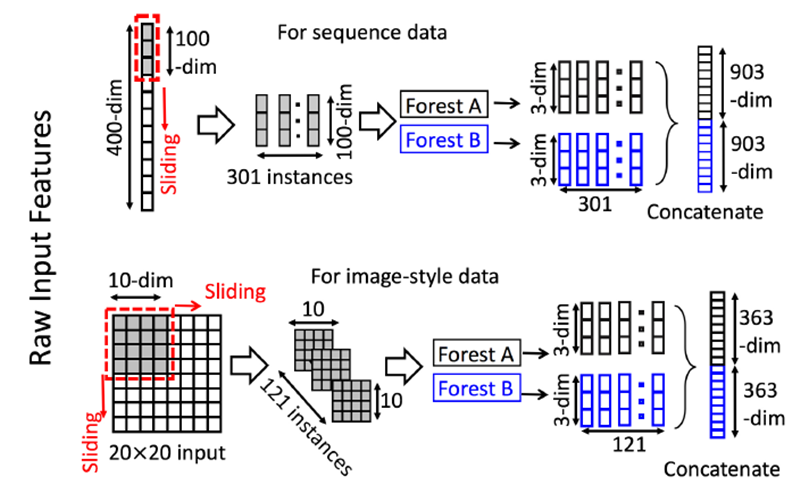• 先输入一个完整的p维样本（p是样本特征的维度），然后通过一个长度为k的采样窗口进行滑动采样，得到s=(p-k)+1个（这个过程就类似于CNN中的卷积核的滑动，这里假设窗口移动的步长为1）k维特征子样本向量；
• 接着每个子样本都用于完全随机森林和普通随机森林的训练，并在每个森林都获得一个长度为c的概率向量（c是分类类别个数，上图中c=3），这样每个森林都产生一个s*c的表征向量，最后把每层的F个森林的结果拼接在一起，得到样本输出。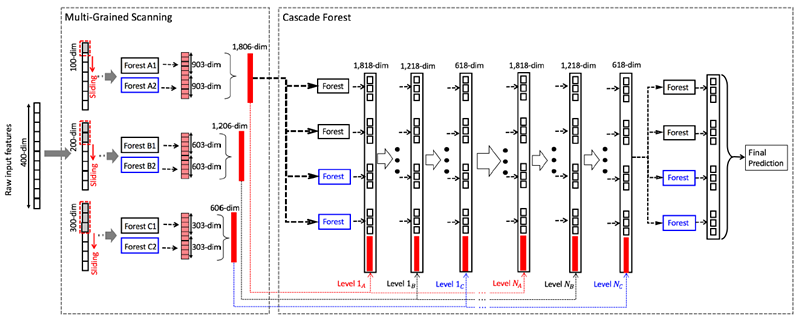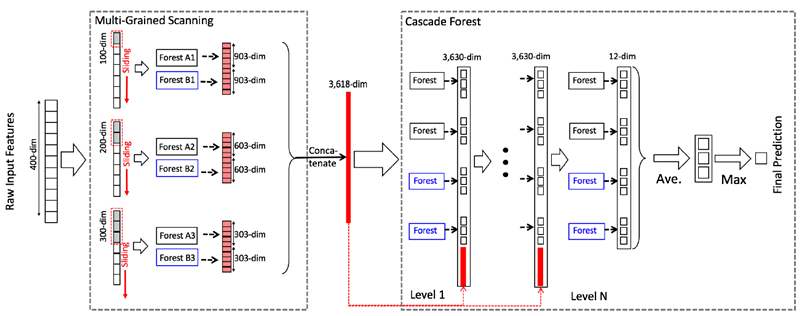## 3.3 小结

• 级联的每层的森林数
• 每个森林的决策树的数量
• 树停止生长的规则

• 多粒度扫描的森林数
• 每个森林的决策树数
• 树的停止生长规则
• 滑动窗口的数量和大小

• 计算开销小，单机就相当于DNN中使用GPU（如果你没有钱购买GPU,可以考虑使用gcForest）
• 模型效果好（论文中[1,2]实验对比了不同模型的效果，gcForest在传统的机器学习，图像，文本，语音上都表现不俗）
• 超参数少，模型对超参数不敏感，一套超参数可以应用到不同的数据集
• 可以适用于不同大小的数据集，模型复杂度可自适用的伸缩
• 每个级联生成使用交叉验证，避免过拟合
• 相对于DNN，gcForest更容易进行理论分析

# 4.gcForest模型的Python实现

GitHub上有两个star比较多的gcForest项目，在参考文献中已经列出，下面我们就使用这两个gcForest的Python模块去尝试使用gcForest模型去解决一些问题，这里要说明的是其中参考文献是官方提供（由gcForest的作者之一Ji Feng维护）的一个Python版本。目前gcForest并没有相对好用的R语言接口，我们已经开发了基于参考文献的R接口包（目前已托管在GitHub和CRAN）。这里我们仅介绍参考文献中的gcForest模块，如果感兴趣，可以自行研究官方提供的gcForest模块（官方模块，集成模型的选择除了随机森林外还可以选择其他的结构像XGBoost，ExtraTreesClassifier LogisticRegression，SGDClassifier等，因此建议使用该模块）。这里仅介绍参考文献中gcForest的模块使用。

Example1:Digits数据集预测

from GCForest import gcForest
from sklearn.model_selection import train_test_split
from sklearn.metrics import accuracy_score

import pandas as pd
import numpy as np

X = digits.data
y = digits.target
X_tr, X_te, y_tr, y_te = train_test_split(X, y, test_size=0.4)

# 注意参数设定
gcf = gcForest(shape_1X=[8,8], window=[4,6], tolerance=0.0, min_samples_mgs=10, min_samples_cascade=7)
gcf.fit(X_tr, y_tr)

# 类别预测
pred_X = gcf.predict(X_te)
print(pred_X)

# 模型评估
accuracy = accuracy_score(y_true=y_te, y_pred=pred_X)
print('gcForest accuracy : {}'.format(accuracy))


Example2:多粒度扫描和级联结构分离

# 模型根据y_tr进行训练
gcf = gcForest(shape_1X=[8,8], window=5, min_samples_mgs=10, min_samples_cascade=7)
X_tr_mgs = gcf.mg_scanning(X_tr, y_tr)

# 回调最后训练的随机森林模型
X_te_mgs = gcf.mg_scanning(X_te)

# 使用多粒度扫描的输出作为级联结构的输入，这里要注意
# 级联结构不能直接返回预测，而是最后一层级联Level的结果
# 因此，需要求平均并且取做大作为预测值

tmp = np.mean(pred_proba, axis=0)
preds = np.argmax(tmp, axis=1)
accuracy_score(y_true=y_te, y_pred=preds)


Example3:去除多粒度扫描的级联结构

gcf = gcForest(tolerance=0.0, min_samples_cascade=20)

tmp = np.mean(pred_proba, axis=0)
preds = np.argmax(tmp, axis=1)
accuracy_score(y_true=y_te, y_pred=preds)


# 5.gcForest模型的R实现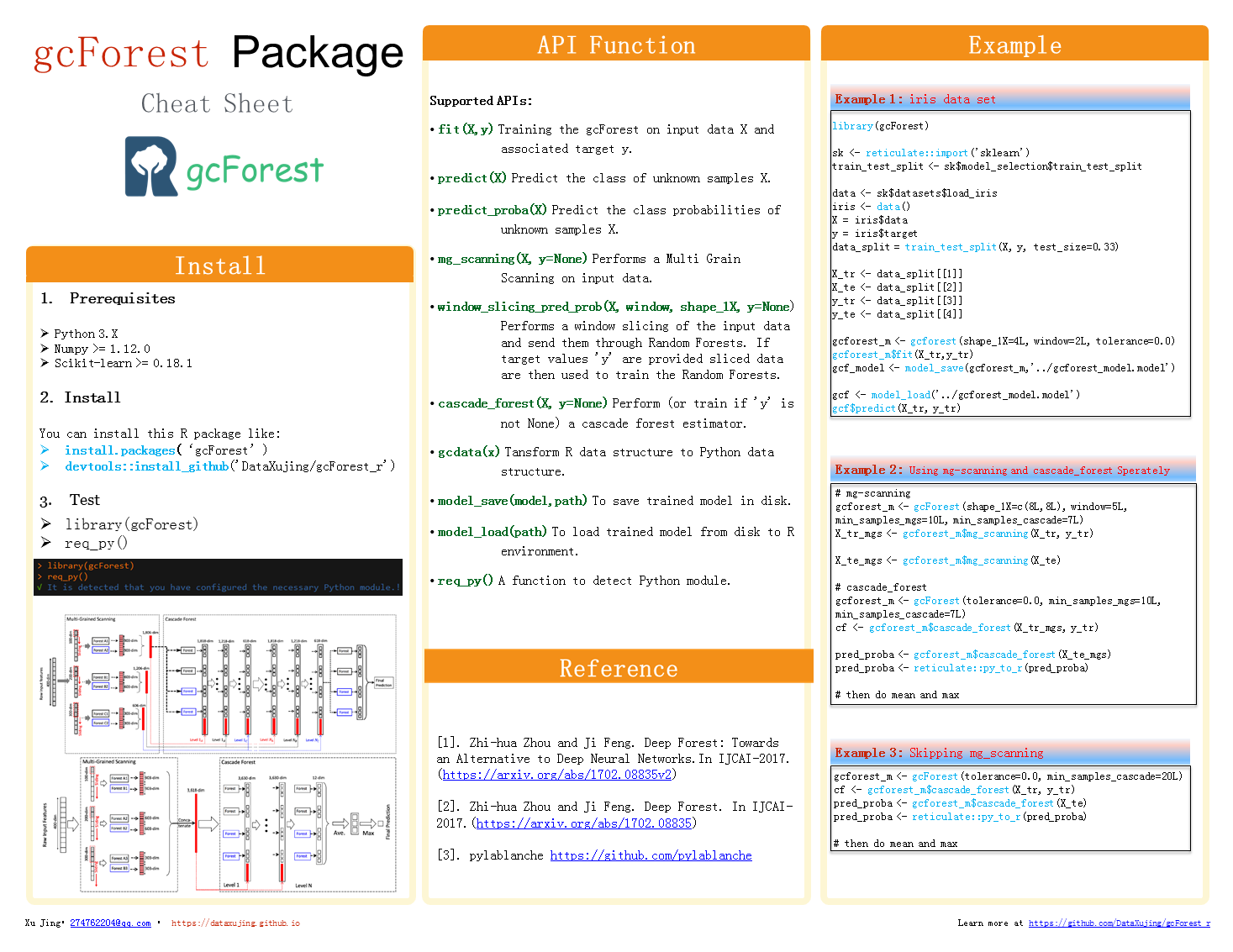Example1:iris数据集预测

# install from CRAN
# install.packages('gcForest')

# install from github
# install.packages("devtools")
# devtools::install_github('DataXujing/gcForest_r')

# 在运行gcForest包前，请确保系统中已经安装了Python3.X及相应的模块包括：
# Numpy >= 1.12.0
# Scikit-learn >= 0.18.1

# 如果你不想手动安装可以通过req_py()函数，进行Python环境的包的监测和安装：
# library(gcForest)
# req_py()

library(gcForest)

sk <- reticulate::import('sklearn')
train_test_split <- sk$model_selection$train_test_split

data <- sk$datasets$load_iris
iris <- data()
X = iris$data y = iris$target
data_split = train_test_split(X, y, test_size=0.33)

X_tr <- data_split[]
X_te <- data_split[]
y_tr <- data_split[]
y_te <- data_split[]

gcforest_m <- gcforest(shape_1X=4L, window=2L, tolerance=0.0)
gcforest_m$fit(X_tr,y_tr) gcf_model <- model_save(gcforest_m,'../gcforest_model.model') gcf <- model_load('../gcforest_model.model') gcf$predict(X_te)


Example2: 多粒度扫描和级联结构分离

# mg-scanning
gcforest_m <- gcForest(shape_1X=c(8L,8L), window=5L, min_samples_mgs=10L, min_samples_cascade=7L)
X_tr_mgs <- gcforest_m$mg_scanning(X_tr, y_tr) X_te_mgs <- gcforest_m$mg_scanning(X_te)

cf <- gcforest_m$cascade_forest(X_tr_mgs, y_tr) pred_proba <- gcforest_m$cascade_forest(X_te_mgs)
pred_proba <- reticulate::py_to_r(pred_proba)

# then do mean and max


# 7.参考文献

. Z.-H. Zhou and J. Feng. Deep Forest: Towards an Alternative to Deep Neural Networks. In IJCAI-2017. (https://arxiv.org/abs/1702.08835v2 )

. Z.-H. Zhou and J. Feng. Deep Forest. In IJCAI-2017. (https://arxiv.org/abs/1702.08835 )

.gcForest v1.1.1(https://github.com/kingfengji/gcForest)

.gcForest version 0.1.6(https://github.com/pylablanche/gcForest)

.gcForest_r(https://github.com/DataXujing/gcForest_r)

.CRAN gcForest(https://CRAN.R-project.org/package=gcForest)